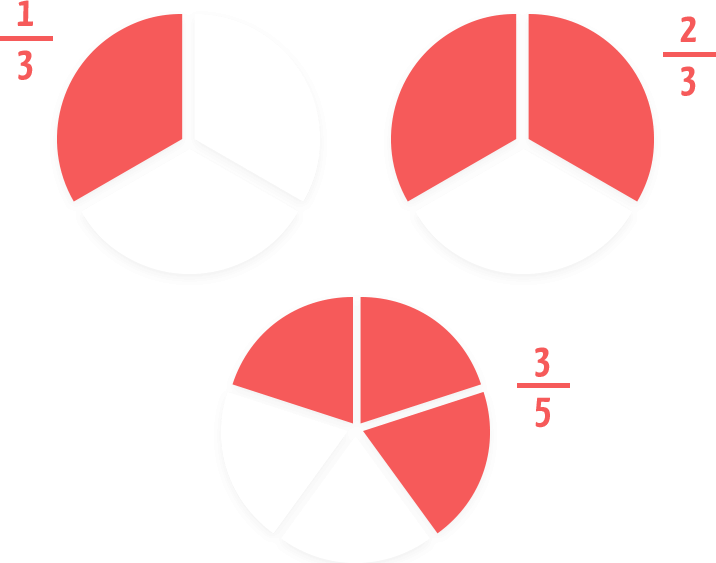# Math Standard CourseLearn English Through Math: Build Core Skills With Math!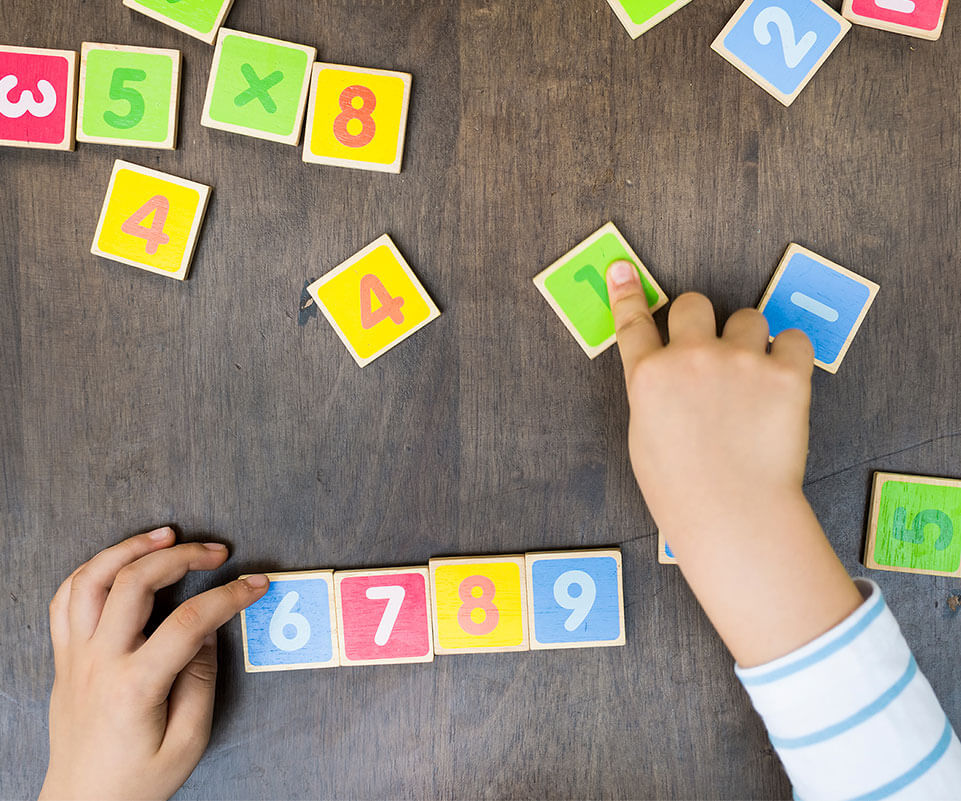#### Math Standard: Learning English Through Math

Our Math Standard course is designed to elevate your child’s ability to verbally express their mathematical knowledge. Lessons go beyond teaching formulas, and challenge students to apply different strategies to solve real world problems. Based on the Common Core Curriculum, the core focus is on strengthening number sense and problem solving skills.These highly motivating and creatively demanding lessons are conducted in English and adjusted to the pace of each individual student.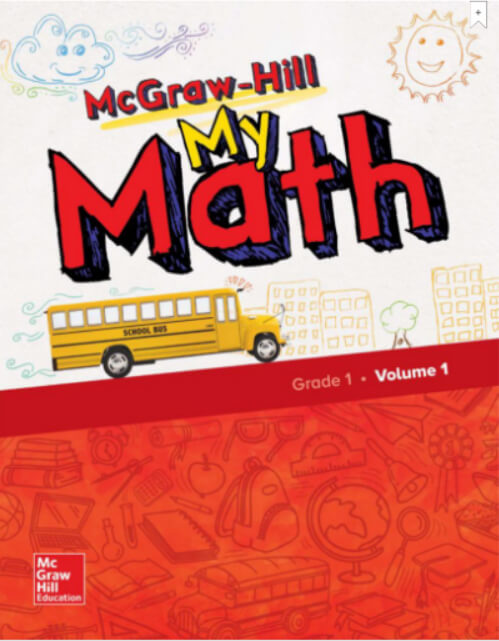The My Math leveled coursebooks teach students how to use tools, encourages students to problem-solve using English vocabulary, and provides your child with additional homework activities.

#### Lesson Pace

At Global Step Academy, you can freely choose the date/time of your lessons, and book them according to your pace. However, we understand that every student has different goals, whether that is becoming able to hold daily conversations or becoming a native speaker. Thus, the lesson pace required is difficult to determine. Please refer to the goal-based, recommended lesson frequencies below.

#### Level Placement and My Math Textbook Fee

Your child's Math Standard level will be determined after the first three lessons. There is a separate fee for textbooks (2,000 yen including tax for the first book, 4,000 yen including tax for the second and subsequent books.) You can pay by GSA coins. You can pay with GSA coins. The amount in coins will be calculated based on your price of additional coins.

#### Beginner Course

Have fun learning math basics in English
In the beginner course, your child will learn the vocabulary and foundational skills needed to solve math problems in English.
Eventually, your child will move up to the intermediate course.

Target Age (approx.)
6-8

Lesson Time
25 mins. / Lesson

Curriculum Level
Kindergarten - Grade 2 (for native speakers)

Coins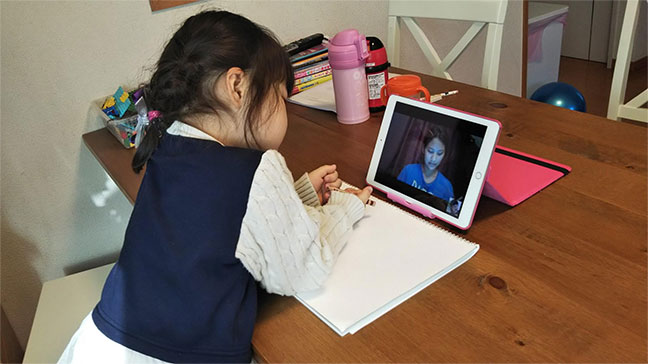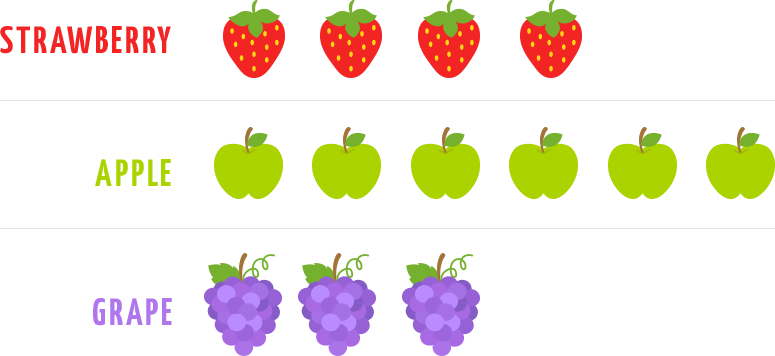#### Lesson Content For Beginner Course

In the beginner course, students will learn basic calculations such as addition and subtraction, as well as place value concepts, while conversing with their teacher in English.

#### Beginner Course Curriculum

• Place value
• Addition and subtraction of 2-digit numbers
• Organizing data and using tables
• Measurements and time
• Plane figures and division (fractions)
• 3-dimensional figures

• Number patterns
• Addition and subtraction of 2-digit numbers
• Value of the thousands place
• Addition and subtraction of 3-digit numbers
• U.S. Money system
• Analyzing data
• Time
• Units: Imperial and metric system
• Geometric forms and divisions (fractions)

#### Intermediate Course

Try out more difficult math problems in English!
In the intermediate course, your child will learn mathematics for upper-class elementary school students in English and analyze number problems in English to deepen their understanding of math concepts and apply the English language in new ways

Target Age (approx.)
8 - 11

Lesson Time
50 min per lesson

Curriculum Level